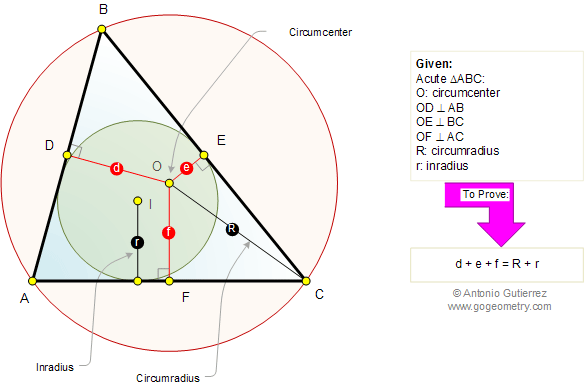# Online Geometry: Carnot's Theorem in an Acute Triangle

In an acute triangle ABC, the sum of the distances from the circumcenter O to the sides, is equal to the sum of the circumradius (R) and the inradius (r).Home | Geometry | Search | Problems | Classical Theorems | Perpendicular Bisector | Email | Post a comment or solution | By Antonio Gutierrez